# NYT-style urban heat island maps

One of my favorite strategies for learning more about data visualization is to try to recreate other work. This is especially true for maps! I recently came across a New York Times article called Summer in the City Is Hot, but Some Neighborhoods Suffer More by Nadja Popovich and Christopher Flavelle. It talks about how urban areas are hotter than non-urban areas on average, but within urban areas there can be a lot of variation. For example areas near parks and water are cooler and industrial parks are hotter.

The article had a lot of really great information, but the most striking thing to me was the maps. They mapped a few different urban areas in the US, including Baltimore, DC, and Albuquerque. I’d encourage you to read it!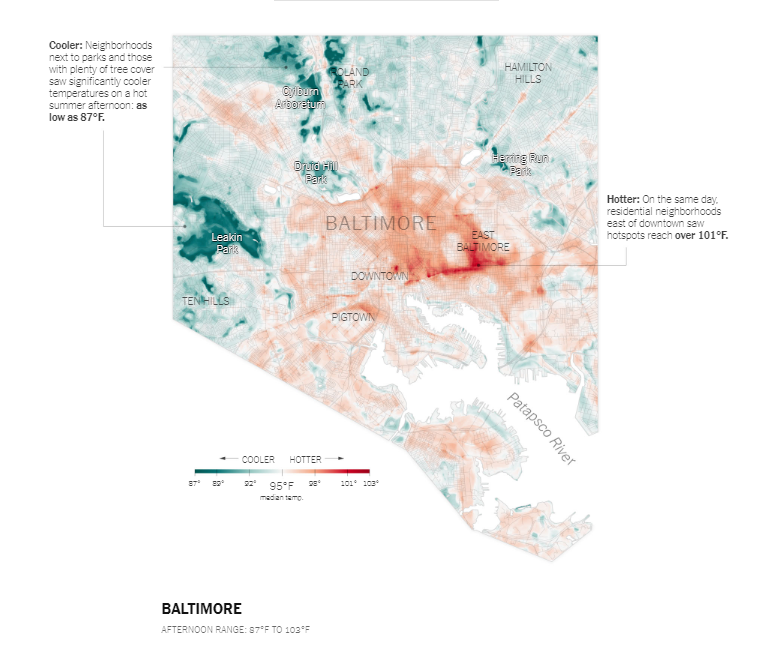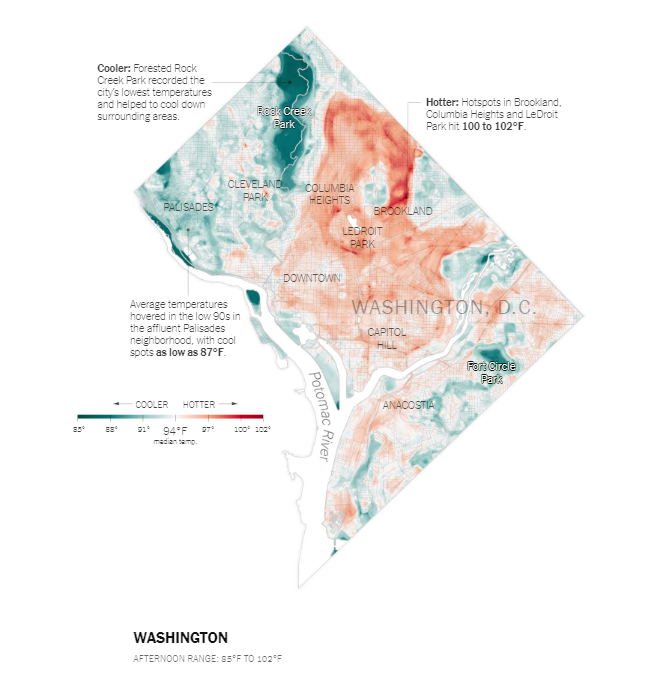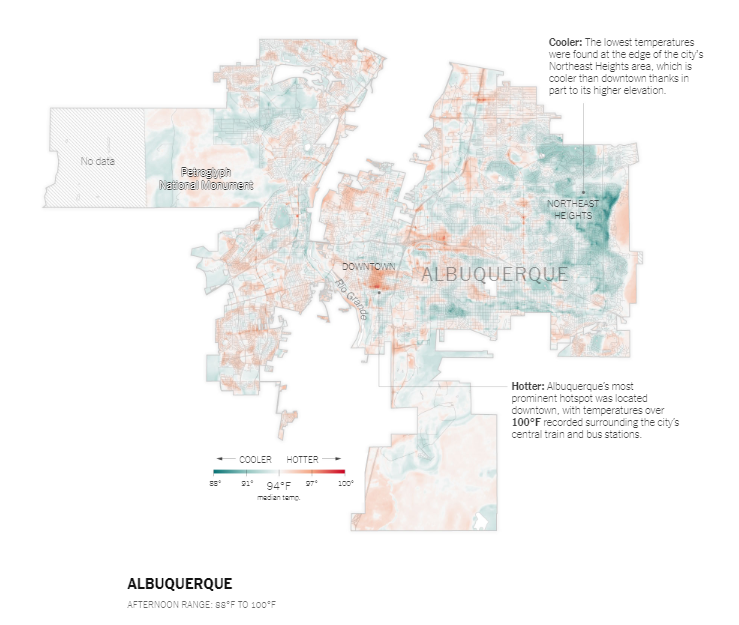Landsat (raster) temperature data for DC was the easiest to find on the city’s open data portal, so that was the map I decided to make. I also wanted to make it completely in R, or as much as I reasonably could. I chose the July 2018 data, but you could chose from any of the available files and use the same code for the map. It would also be interesting to compare patterns over time!

library(raster) # reading in and wrangling landsat data
library(sf) # reading in and wrangling contextual data
library(tidyverse)
# devtools::install_github("clauswilke/ggtext")
library(ggtext) # text in plots
library(showtext) # more fonts
font_add_google("Lato", regular.wt = 300, bold.wt = 700) # I like using Lato for data viz (and everything else...). Open sans is also great for web viewing.


To make the maps, I started by using base R plotting but eventually moved to ggplot2, mostly for easier text integration.

landsat_dc_july18 <- raster("data/LST_F_20180708.tif") # saved the downloaded files in a data/ folder

# water features in the city
st_transform(st_crs(landsat_dc_july18)) # use the same coordinate reference system as the landsat data


Then we can get a nice summary of the landsat data just with raster functions.

landsat_dc_july18 # gives info like extent, crs, min/max

## class       : RasterLayer
## dimensions  : 768, 623, 478464  (nrow, ncol, ncell)
## resolution  : 30, 30  (x, y)
## extent      : 389448.6, 408138.6, 124698.5, 147738.5  (xmin, xmax, ymin, ymax)
## coord. ref. : +proj=lcc +lat_1=39.45 +lat_2=38.3 +lat_0=37.66666666666666 +lon_0=-77 +x_0=400000 +y_0=0 +datum=NAD83 +units=m +no_defs +ellps=GRS80 +towgs84=0,0,0
## data source : C:/Users/katie/Documents/heat_islands/data/LST_F_20180708.tif
## names       : LST_F_20180708
## values      : 72.48885, 106.6179  (min, max)

summary(landsat_dc_july18) # gives distribution summary based on a sample

##         LST_F_20180708
## Min.          72.48885
## 1st Qu.       82.26950
## Median        88.02995
## 3rd Qu.       91.33167
## Max.         105.87772
## NA's           0.00000


From this we can see that the temperature ranges from 72 to 106 F with a median of 88 F and there are no NAs.

To plot this data with ggplot2 I first converted the raster values to a dataframe in two steps.

temp_spdf <- as(landsat_dc_july18, "SpatialPointsDataFrame") # create spatialpoints dataframe
temp_df <- as_tibble(temp_spdf) # convert that to a plain tibble
colnames(temp_df) <- c("value", "x", "y")


Then I also converted the water data to an sp object in order to use the coord_equal() function in ggplot2, more on that later.

water_sp <- as(water, "Spatial")


At this point we can make a “minimum viable product” map.

ggplot() +
geom_raster(data = temp_df, aes(x = x, y = y,  fill = value), interpolate = TRUE) +
geom_polygon(data = water_sp, aes(x = long, y = lat, group = group), color = "gray90", fill = "white") +
coord_equal() + # make sure that the x and y axis are comparable
theme_minimal()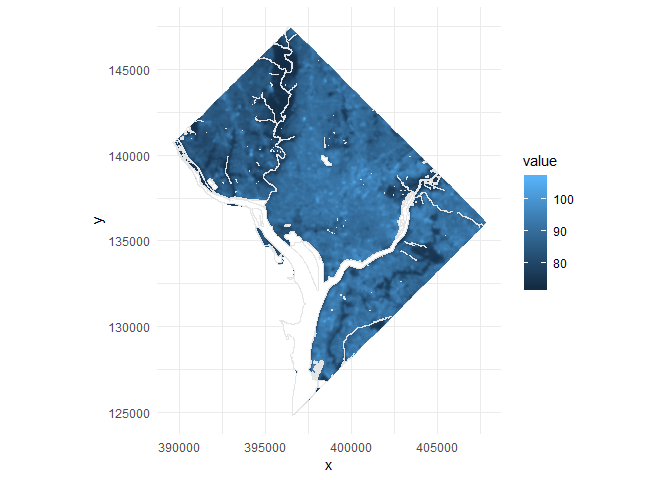It’s hard to see the patterns with this color scheme, though. A diverging color scheme would highlight relative low and high values. I started with generating color schemes using the Chroma.js Color Palette Helper but I ended up using the R package rcartocolor which integrates the Carto color schemes.

On the Carto site you can test out different color schemes. I wanted to keep the idea of the color scheme from the original (cool and warm colors mapped to cool and warm temperatures), but wanted to change the hues slightly. I decided to go with the teal rose color scheme.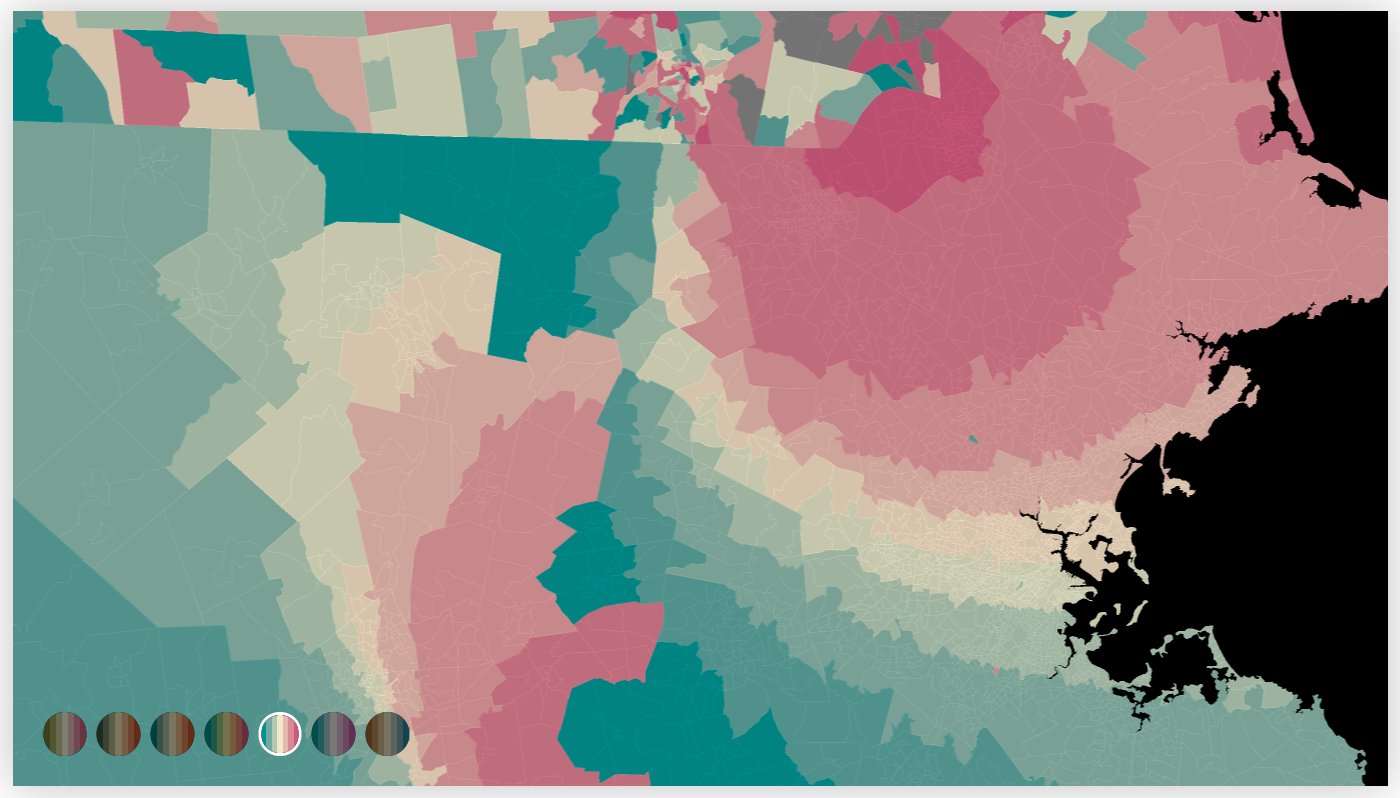I added that color scheme to my viz and set the breaks on the legend to the minimum, median, and maximum.

ggplot() +
geom_raster(data = temp_df, aes(x = x, y = y,  fill = value), interpolate = TRUE) +
geom_polygon(data = water_sp, aes(x = long, y = lat, group = group), color = "gray90", fill = "white") +
theme_minimal() +
coord_equal() +
scale_fill_gradientn(colors = rcartocolor::carto_pal(name = "TealRose", n = 7), breaks = c(72.5, 88, 106.6), labels = c("72", "88", "106"), name = "")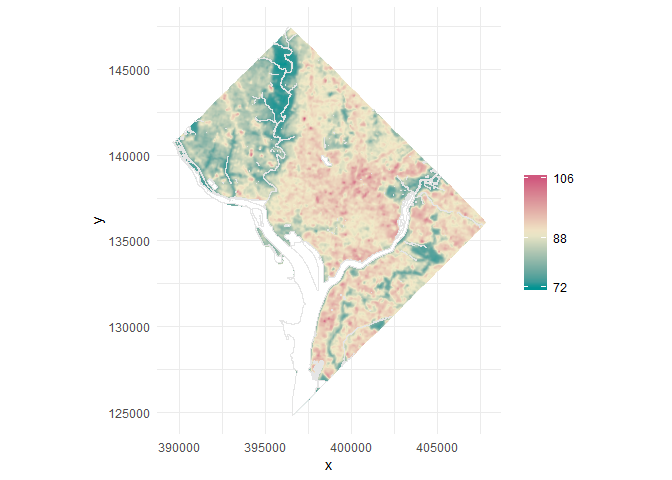Now we can really start to see the differentiation across the city. The next step is to add the text neighborhood labels and descriptions in the margins. To do this I used the ggtext package which allows for markdown formatting, so it was easy to have bold characters. I created a tibble with x/y coordinates and labels. To figure out the coordinates I used the axis ticks as a starting point and then trial and error from there. An easier way to get neighborhood labels could have been to have a neighborhood shapefile and use centroids from that. This method is pretty inefficient but it worked well for me this time.

labels <- tibble(x = c(396000, 395900, 390500,
399700, 396000, 399700, 399200, 389070, 390000),
y = c(138300, 136600, 140000,
133000, 140000, 140500, 146400, 147000, 130100),
lab = c("W A S H I N G T O N", "downtown", "palisades", "anacostia", "columbia<br> heights", "brookland", "**Hotter:** Hotspots in<br>Brookland, Columbia<br>Heights, and LeDroit<br>Park hit **100 to 106 F**", "**Cooler:** Forested Rock<br>Creek Park recorded the<br>city's lowest temperatures<br>and helped to cool down<br>surrounding areas", "**Urban green spaces**<br>are an invaluable<br>resources for cooling<br>urban neighborhoods.<br>They help promote<br>walkability and improve<br>quality of living. Even a<br>few trees help!"))


I can then add them to the map with geom_richtext(). Note that I set fig.width=14 in my chunk options in order to get the spacing right.

ggplot() +
geom_raster(data = temp_df, aes(x = x, y = y,  fill = value), interpolate = TRUE) +
geom_polygon(data = water_sp, aes(x = long, y = lat, group = group), color = "gray90", fill = "white") +
geom_richtext(data = labels, aes(x = x, y = y, label = lab), fill = NA, label.color = NA, # remove background and outline
label.padding = grid::unit(rep(0, 4), "pt"),  color = "#656c78", hjust = 0)+
theme_minimal() +
coord_equal() +
scale_fill_gradientn(colors = rcartocolor::carto_pal(name = "TealRose", n = 7), breaks = c(72.5, 88, 106.6), labels = c("72", "88", "106"), name = "")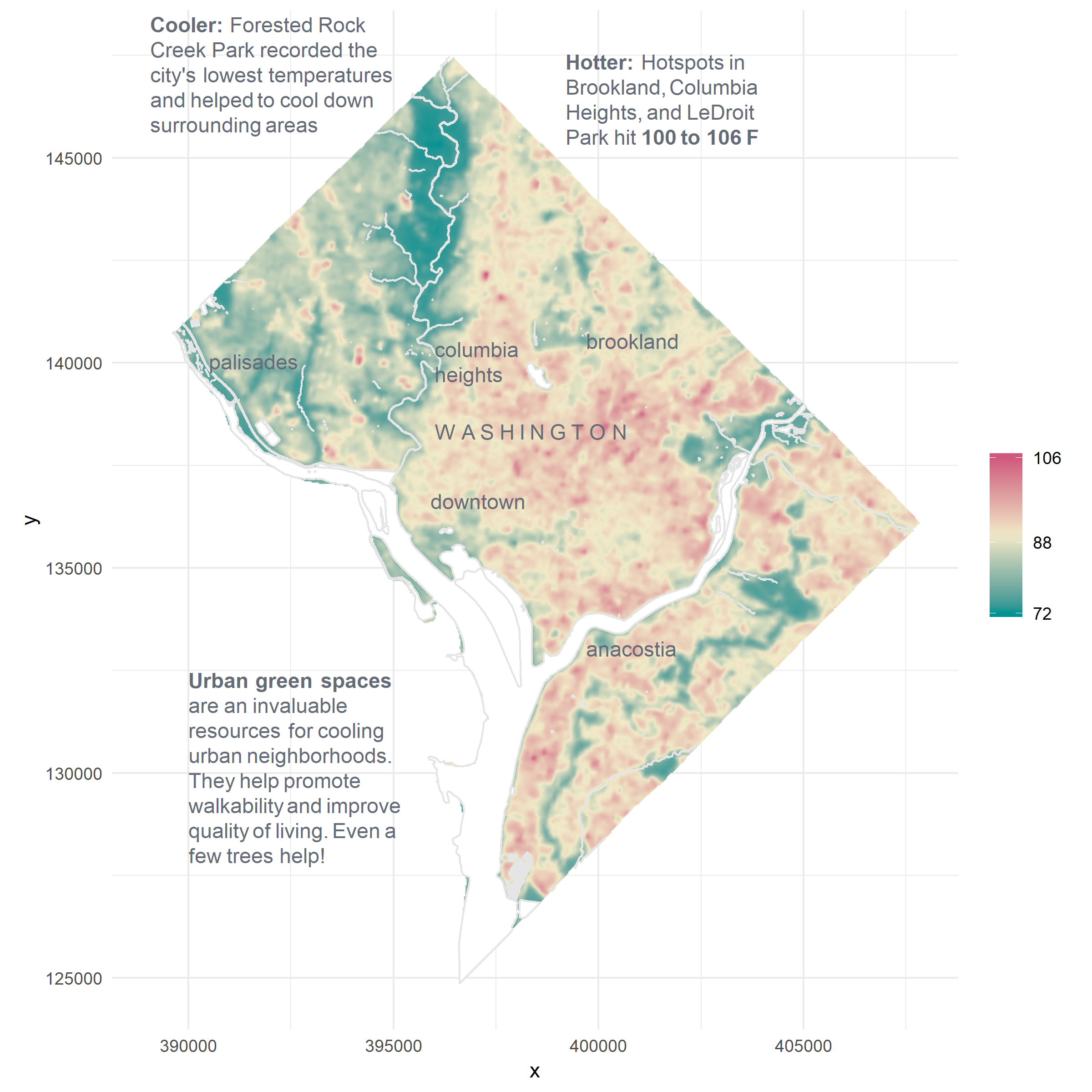Now the only things left to do are cleaning up the plot a bit and reformatting the legend.

ggplot() +
geom_raster(data = temp_df, aes(x = x, y = y,  fill = value), interpolate = TRUE) +
geom_polygon(data = water_sp, aes(x = long, y = lat, group = group), color = "gray90", fill = "white") +
geom_richtext(data = labels, aes(x = x, y = y, label = lab), fill = NA, label.color = NA, # remove background and outline
label.padding = grid::unit(rep(0, 4), "pt"),  color = "#656c78", hjust = 0)+
theme_minimal() +
coord_equal() +
scale_fill_gradientn(colors = rcartocolor::carto_pal(name = "TealRose", n = 7), breaks = c(72.5, 88, 106.6), labels = c("72 F", "88", "106"), name = "") +
theme(legend.position = "bottom",
axis.text = element_blank(),
axis.title = element_blank(),
panel.grid = element_line("transparent"),
text = element_text(color = "#656c78", family = "Lato"),
plot.caption = element_text(hjust = 0)) +
guides(fill = guide_colourbar(barheight = 0.3, barwidth = 20, direction = "horizontal", ticks = FALSE)) +
labs(caption = "July 8, 2018\nSource: DC Open Data")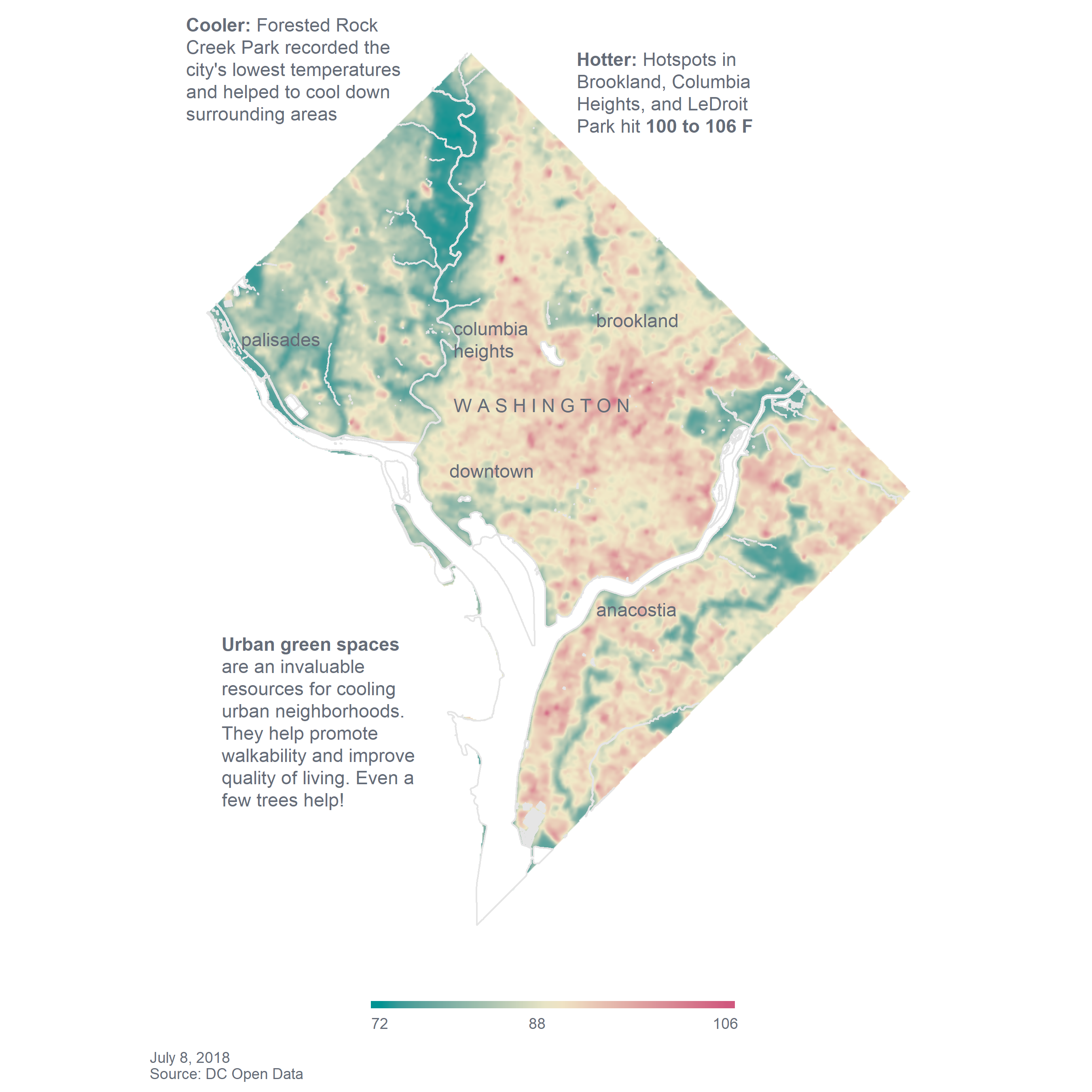And now we have our map!

I was also curious about where the hottest areas in the city were so I mapped everywhere that reached at least 95 F.

over95 <- temp_df %>%
filter(value >=95) # where the value is 95 or greater

labels2 <- tibble(x = c(396000, 395900, 390500,
399700, 396000, 399700, 399200, 389000),
y = c(138300, 136600, 140000,
133000, 140000, 140500, 146400, 132500),
lab = c("W A S H I N G T O N", "downtown", "palisades", "anacostia", "columbia<br> heights", "brookland", "The locations in **red** all<br>reached at least **95 F** while<br> the median was only **88 F**", "The hotter areas are primarily<br>in the NE quadrant which has<br>historically been more industrial"))

ggplot() +
geom_raster(data = temp_df, aes(x = x, y = y), fill = "gray90", interpolate = TRUE) +
geom_raster(data = over95, aes(x = x, y = y), fill = "#d0587e") +
geom_polygon(data = water_sp, aes(x = long, y = lat, group = group), color = "gray90", fill = "white") +
geom_richtext(data = labels2, aes(x = x, y = y, label = lab), fill = NA, label.color = NA, # remove background and outline
label.padding = grid::unit(rep(0, 4), "pt"),  color = "#656c78", hjust = 0)+
theme_minimal() +
coord_equal() +
scale_fill_gradientn(colors = rcartocolor::carto_pal(name = "TealRose", n = 7), breaks = c(72.5, 88, 106.6), labels = c("72", "88", "106"), name = "") +
theme(legend.position = "bottom",
axis.text = element_blank(),
axis.title = element_blank(),
panel.grid = element_line("transparent"),
text = element_text(color = "#656c78", family = "Lato"),
plot.caption = element_text(hjust = 0)) +
guides(fill = guide_colourbar(barheight = 0.3, barwidth = 15, direction = "horizontal", ticks = FALSE)) +
labs(caption = "July 8, 2018\nSource: DC Open Data")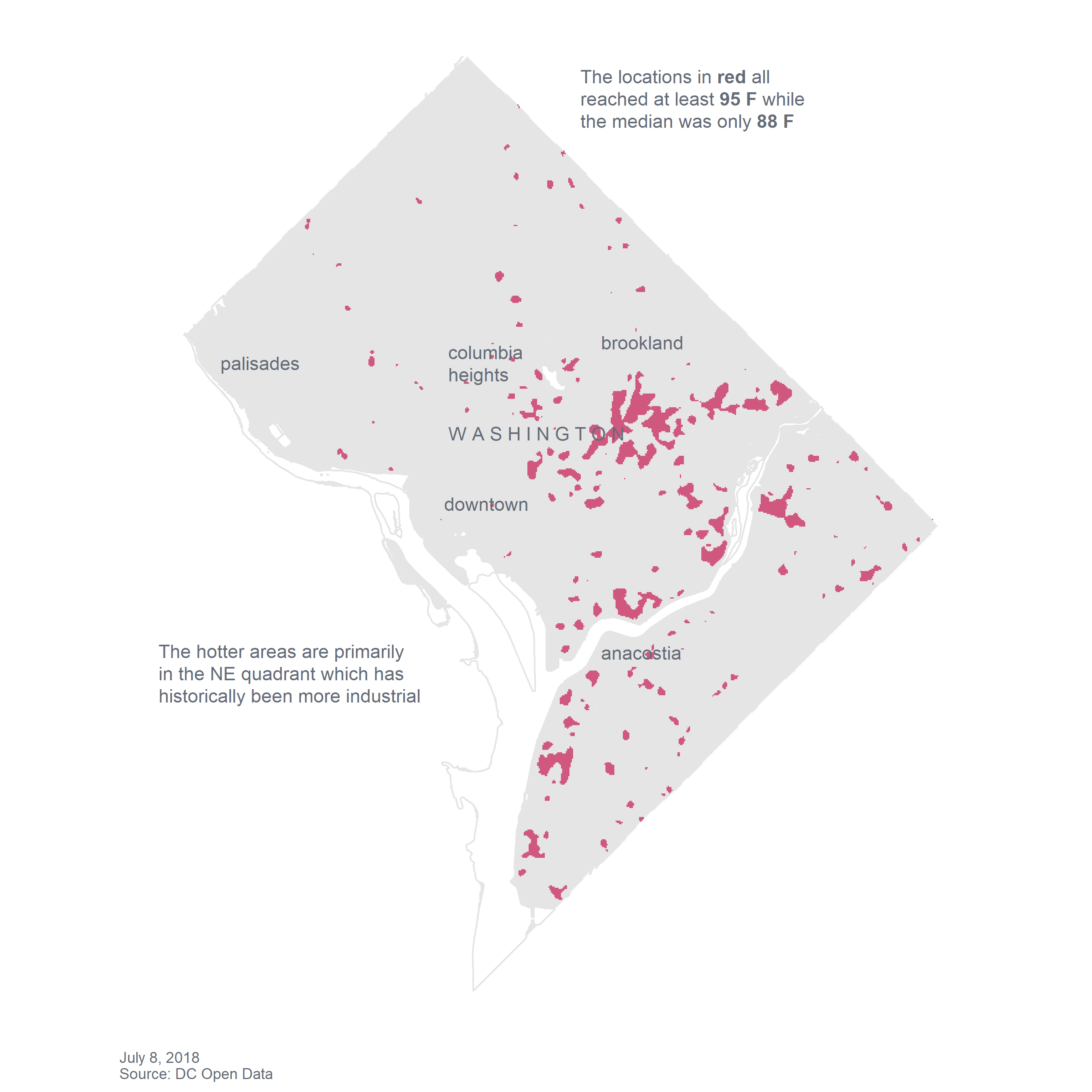And all of the areas that were less than 80 F.

under80 <- temp_df %>%
filter(value <= 80)

labels3 <- tibble(x = c(396000, 395900, 390500,
399700, 396000, 399700, 399200, 389000),
y = c(138300, 136600, 140000,
133000, 140000, 140500, 146400, 132100),
lab = c("W A S H I N G T O N", "downtown", "palisades", "anacostia", "columbia<br> heights", "brookland", "The locations in **green** all<br>reached only **80 F** while<br> the median was **88 F**", "The cooler areas are primarily<br>in parks like Rock Creek Park,<br>the National Arboretum, and<br>Fort Circle Park"))

ggplot() +
geom_raster(data = temp_df, aes(x = x, y = y), fill = "gray90", interpolate = TRUE) +
geom_raster(data = under80, aes(x = x, y = y), fill = "#009392") +
geom_polygon(data = water_sp, aes(x = long, y = lat, group = group), color = "gray90", fill = "white") +
geom_richtext(data = labels3, aes(x = x, y = y, label = lab), fill = NA, label.color = NA, # remove background and outline
label.padding = grid::unit(rep(0, 4), "pt"),  color = "#656c78", hjust = 0)+
theme_minimal() +
coord_equal() +
theme(legend.position = "bottom",
axis.text = element_blank(),
axis.title = element_blank(),
panel.grid = element_line("transparent"),
text = element_text(color = "#656c78", family = "Lato"),
plot.caption = element_text(hjust = 0)) +
guides(fill = guide_colourbar(barheight = 0.3, barwidth = 15, direction = "horizontal", ticks = FALSE)) +
labs(caption = "July 8, 2018\nSource: DC Open Data")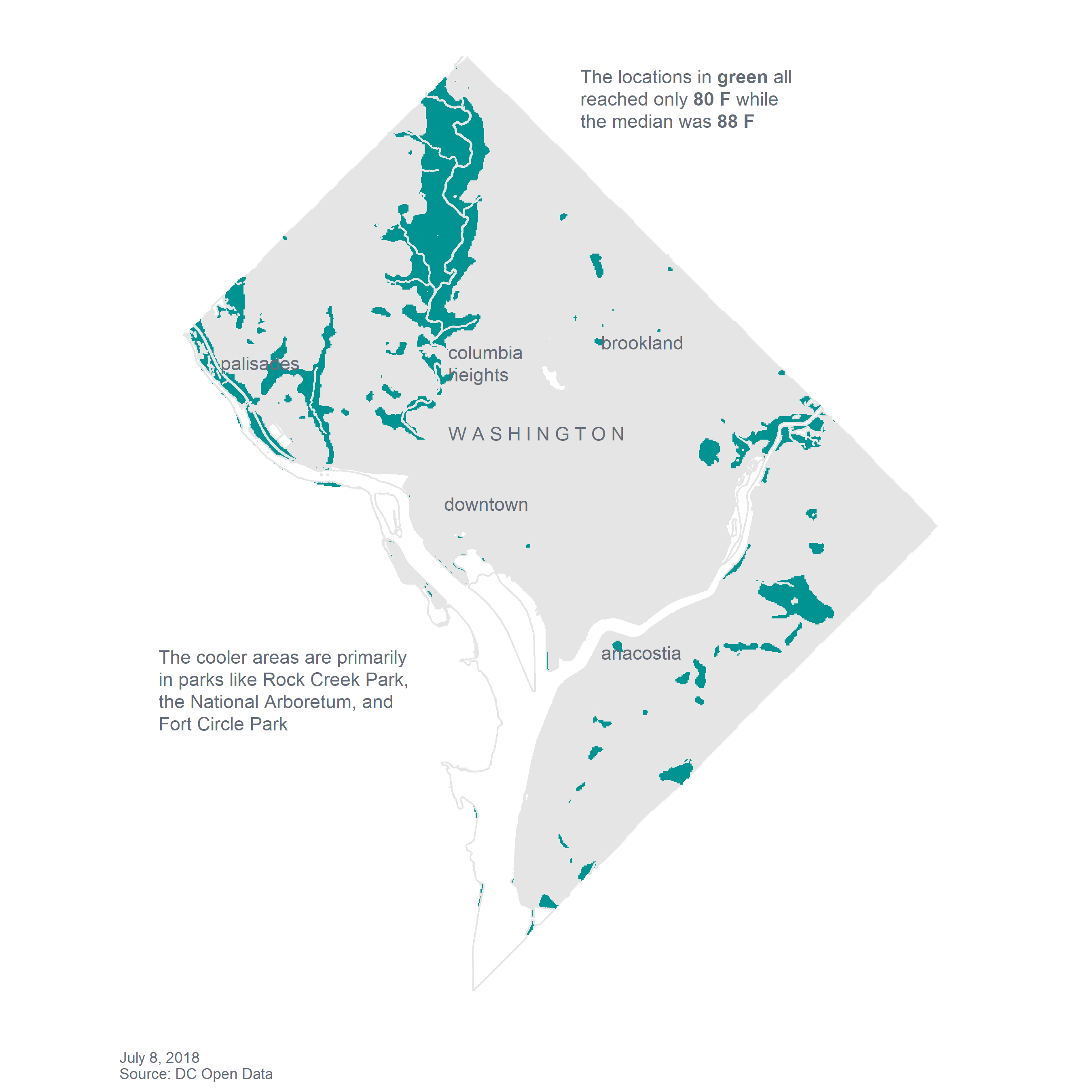I said I’d get back to why I use sp data structures for the water shapefile. coord_equal() seems to not work with sf, so instead of figuring out why I just went ahead with what I knew would work. Albeit not a great strategy long-term.